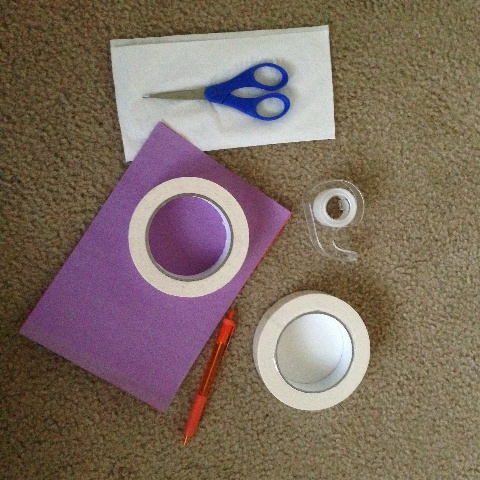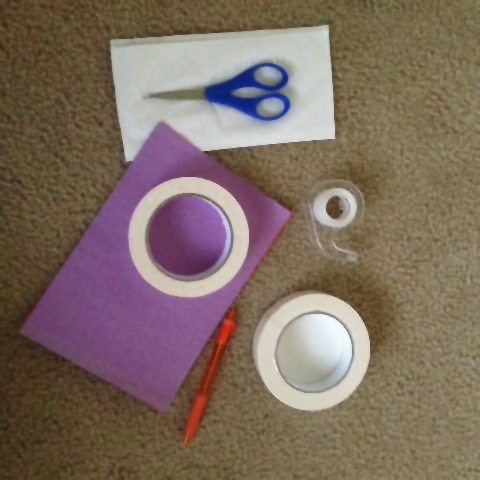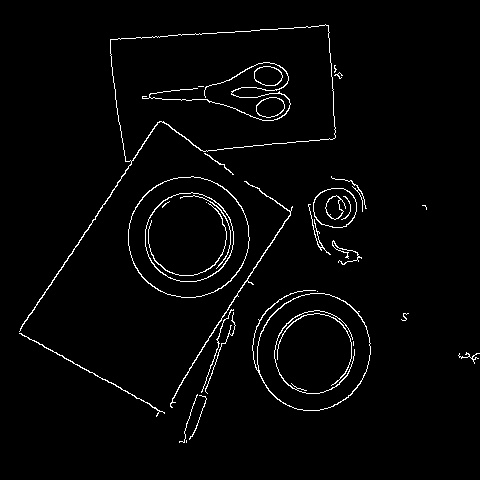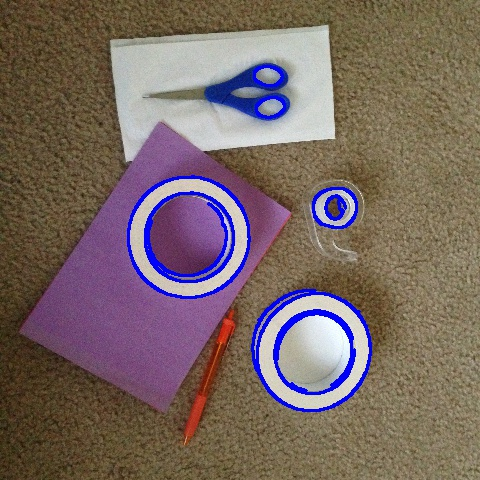# Detecting circular shapes using contours

Last weekend, I was in a very creative mood. Did some origami. It was fun! Just after I completed my work, I had the papers, tapes and scissors lying around. Well, I thought, ‘why not teach my robot to learn few shapes.’ And I decided to go with circular shapes.

To do that, I started taking a few pictures of the objects. After some random clicks, I took up this picture to do the object detection based on circular shapes.

## Raw images:```rawImage = cv2.imread('rawImage.jpg')
cv2.imshow('Original Image', rawImage)
cv2.waitKey(0)
```

Why did I pick this image? Well.. This image has few different objects. It has a noisy background. It has objects of various shapes, and yet, there are few circular shapes that can be found. I think this will serve as a good example for teaching my robot.

Before we start finding the objects, let’s clean it a bit. By cleaning I mean reducing the background noise, highlight the region we are interested in, and then go about finding objects. This step is called preprocessing the image.

## Bilateral Filtering

What is this filter? Bilateral filtering forms a very good way to preserve edges. It is a non-linear filter and helps reduce noise. The concept of this filter is that, at each pixel, its value is substituted by the average of the neighbourhood pixels. That gives the smooth effect when this filter is applied. In our image, we see that the background is not very even. This filter will help to even out the surface, preserving the edges. OpenCV provides a function to implement bilateral filter. The parameters used are: the image, window size for averaging the neighbour, sigmaColor(Sigma value in the color space. This says that the farther colors in the pixel neighbour will be mixed together. The larger the value, usually greater that 150, the greater is the effect), sigmaSpace(Sigma value in coordinate space. Larger value says that farther pixels will influence the current pixel). Code below demonstrates a bilateral filter.

```bilateral_filtered_image = cv2.bilateralFilter(rawImage, 5, 175, 175)
cv2.imshow('Bilateral', bilateral_filtered_image)
cv2.waitKey(0)
```## Edge Detection

We applied a bilateral filter to preserve the edges. Now, it’s time to detect the edges. We will use Canny edge detector to detect edges in the image. By tracing the edges, we are extracting features of the image. This detector uses two threshold values. The Canny algorithm uses the first threshold to find the fine links and the second threshold to find strong edges. The algorithm uses Gaussian filter, intensity gradient and non-maxima suppression in the process of finding the edges. OpenCV provides a function to implement edge detection using Canny algorithm. It takes 3 parameters: image, lower threshold and upper threshold.

On the bilateral filtered image, we will apply canny filter as below:

```edge_detected_image = cv2.Canny(bilateral_filtered_image, 75, 200)
cv2.imshow('Edge', edge_detected_image)
cv2.waitKey(0)
```

This code gives the following output:## Finding contours

In my previous blog about Object detection, we learnt the concept of contours. These are mathematical structures with shapes that are formed by joining the points covering an area of similar intensity or color. We are going to use this on the edges detected in the pre processing step. This will help in finding objects(contours) in the image. OpenCV has provides the below function to find contours.

```_, contours, _= cv2.findContours(edge_detected_image, cv2.RETR_TREE, cv2.CHAIN_APPROX_SIMPLE)
```

The above code gives a list of contours as output. We have lots of objects as contours in this list. We need to filter them by finding relevant objects, here, with particular shape.

## Focus on finding the circular shapes

This is the section where we are going to find circular objects. The concept that we are going to use is, approxPolyDP. What does this do? Well, this function helps to find what kind of polygon is the contour. How? It gives the output as number of vertices for the polygon. For eg., a square contour will give the output as 4 points, pentagon as 5 points and so on. Circular objects will have higher number of points. Here, we see that depending on the type of objects in the image, polygons with greater than 8 vertices form curvier shapes, here circle and ellipse.

If we carefully observe the function for approxPolyDP, we see that it calculates the percentage of arcLength or perimeter of the contour. Approximates the contour with that information. The higher the percentage, the lower the number of vertices. We are therefore using a very low percentage to find details of the contour. After getting that information, we also find the area of the contour. This is done in order to eliminate very small objects. Below code explains the filtering process. Once that is done, we have the required circular shapes.

```contour_list = []
for contour in contours:
approx = cv2.approxPolyDP(contour,0.01*cv2.arcLength(contour,True),True)
area = cv2.contourArea(contour)
if ((len(approx) > 8) & (area > 30) ):
contour_list.append(contour)
```

## Displaying the results

Let us display the objects detected in the above process:

```cv2.drawContours(rawImage, contour_list,  -1, (255,0,0), 2)
cv2.imshow('Objects Detected',rawImage)
cv2.waitKey(0)
```The complete source code can be found below:

```import cv2

cv2.imshow('Original Image', raw_image)
cv2.waitKey(0)

bilateral_filtered_image = cv2.bilateralFilter(raw_image, 5, 175, 175)
cv2.imshow('Bilateral', bilateral_filtered_image)
cv2.waitKey(0)

edge_detected_image = cv2.Canny(bilateral_filtered_image, 75, 200)
cv2.imshow('Edge', edge_detected_image)
cv2.waitKey(0)

_, contours, hierarchy = cv2.findContours(edge_detected_image, cv2.RETR_TREE, cv2.CHAIN_APPROX_SIMPLE)

contour_list = []
for contour in contours:
approx = cv2.approxPolyDP(contour,0.01*cv2.arcLength(contour,True),True)
area = cv2.contourArea(contour)
if ((len(approx) > 8) & (len(approx) < 23) & (area > 30) ):
contour_list.append(contour)

cv2.drawContours(raw_image, contour_list,  -1, (255,0,0), 2)
cv2.imshow('Objects Detected',raw_image)
cv2.waitKey(0)
```

Looks like we could recognize circular shapes, from being a perfect circle to being an ellipse. That’s awesome. approxPolyDP serves very well in determining the type of polygon a figure is. This can be used to determine several shapes, not just circular.

We have determined shapes using on of the ways of shape detection. Next time, we shall see how my robot tries to track objects by the change in their orientation. This would involve, detecting the object and then tracing it through several frames. Till then have a great time.

I hope you found this useful. Please share any tips to make this better.